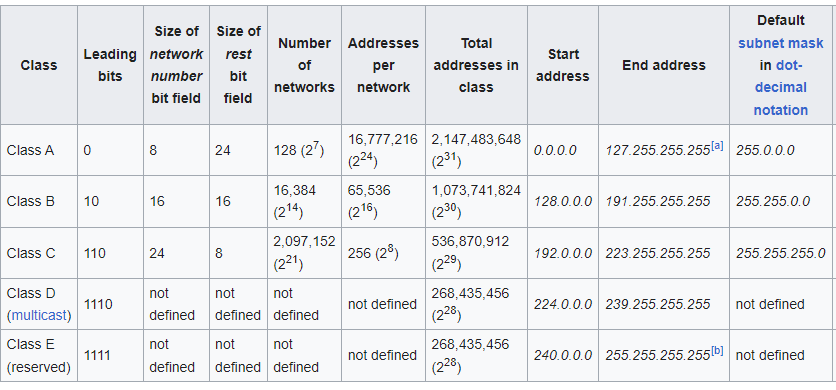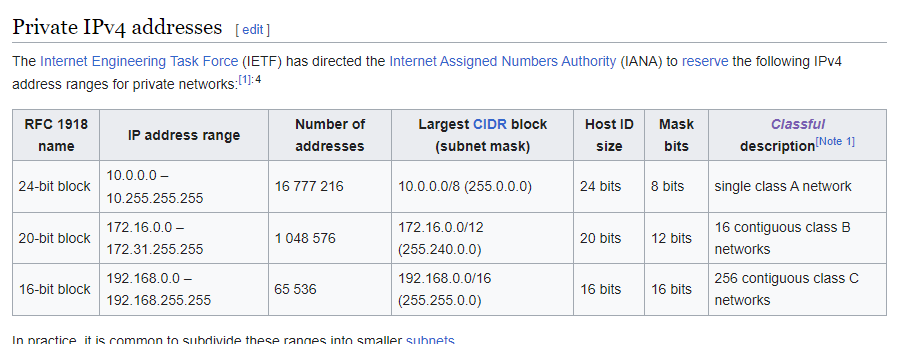## Networking contd

### Size of network

• number of devices that can be connected to the network i.e. number of hosts/devices that can be connected in a network
``````ip: 192.168.100.111
sm: 255.255.255.0

nid: 192.168.100
hid: 111

with in a network
nid = fixed = 3 octets
hid = variable = 1 octet = 8 bits => 2^8 hosts => 256
nid = 192.168.100.0
i.e
size of network => 2^ (host id bits) - 2
=> 2^8 -2
=> 254

ip: 10.100.102.32
sm: 255.255.0.0
nid = 2 octets
hid = 2 octets = 16 bits
size => 2^16 - 2
=> 65536 - 2
=> 65534
``````
• In every network we can use two ip addresses

• 0: this represents network id
• To create networks of different sizes, we have two approaches

• Classful networking• Classless interdomain routing (CIDR)
• CIDR Approach
``````ip: 192.168.100.111
sm: 11111111.11111111.11111111.00000000

nid => number of consecutive 1's = 24 (fixed)
hid => 8 (number of consecutive 0's)

for= 2^ (hid bits) - 2
= 2^8 -2 = 254

ip: 192.168.100.111
sm: 11111111.11111111.11111110.00000000

size = 2^9 - 2 = 512 - 2 = 510
``````
• I have 2000 devices what should be by subnet mask
``````2^n-2 ~= 2000

2^n ~ = 2000
n = 11

sm: 11111111.11111111.11111000.00000000
255.255.248.0
``````
• I have 12000 devices what should be my subnet mask
``````
2^n - 2 ~= 1,20,000
n = 17 (number of zeros)
N = 32 - 17 = 15
sm: 11111111.11111110.00000000.00000000
255.254.0.0
``````
• I have 27 devices what should be my subnet mask
``````2^n -2 ~= 27
n = 5
N = 32-5 = 27

sm: 11111111.11111111.11111111.11100000
255.255.255.224

``````
• I have 2000000 devices what should be my subnet mask
``````2 ^ n -2 ~= 2000000
n = 21
N = 32 - 21 = 11
sm: 11111111.11100000.00000000.00000000
255.224.0.0
``````
• public network: This is an ip accessible from internet and private network: These are reserved for private networking• CIDR Notation
``````x.x.x.x/N
``````
• Expand CIDR
• Sample 1
``````### sample 1
192.168.0.0/24
N = 24
n = 32 -24 = 8
ip:      192.     168.       0.       0
sm: 11111111.11111111.11111111.00000000
start: 192.168.0.00000000 => 192.168.0.0
end:   192.168.0.11111111 => 192.168.0.255
``````
• sample 2
``````cidr: 10.0.0.0/11
N = 11 (number of ones)
n = 32 -11 = 21
sm: 11111111.11100000.00000000.00000000

ip:       10.       0.       0.       0
sm: 11111111.11100000.00000000.00000000
range: 10.000xxxxx.xxxxxxxx.xxxxxxxx
start: 10.00000000.00000000.00000000 = 10.0.0.0
end:   10.00011111.11111111.11111111 => 10.31.255.255

``````
• sample 3
``````cidr: 172.16.0.0/12
N = 12
n = 32 -12 = 20

ip:       172.      16.       0.       0
sm:  11111111.11110000.00000000.00000000
ip:        172.00010000.00000000.00000000
range: 172.0001xxxx.xxxxxxxx.xxxxxxxx
start: 172.00010000.00000000.00000000 => 172.16.0.0
end: 172.00011111.11111111.11111111 => 172.31.255.255

``````
• sample 4
``````192.168.128.0/22
N = 22
n = 32 - 22 = 10
sm: 11111111.11111111.11111100.00000000
ip:      192.     168.100000xx.xxxxxxxx
start:   192.168.128.0
end:     192.168.131.255
``````
• Exercises:
``````10.132.0.0/23
192.168.0.128/27
172.16.0.0/21
``````

This site uses Akismet to reduce spam. Learn how your comment data is processed.